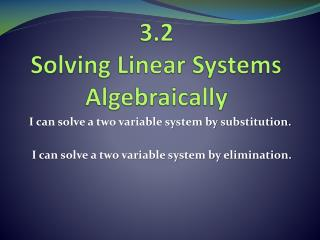DownloadDownload Presentation3.2 Solving Linear Systems Algebraically

# 3.2 Solving Linear Systems Algebraically

Download Presentation## 3.2 Solving Linear Systems Algebraically

- - - - - - - - - - - - - - - - - - - - - - - - - - - E N D - - - - - - - - - - - - - - - - - - - - - - - - - - -
##### Presentation Transcript

1. 3.2Solving Linear Systems Algebraically I can solve a two variable system by substitution. I can solve a two variable system by elimination.

2. The Substitution Method • Step 1: • Solve one of the equations for one of its variables. • Step 2: • Substitute the expression from Step 1 into the other equation and solve for the other variable. • Step 3: • Substitute the value from Step 2 into the equation from Step 1 and solve.

3. Which equation is easiest to get a variable? Example 1: Substitution 3x + 4y = -4 x + 2y = 2 • Step 1: solve for a variable x + 2y = 2 -2y -2y x = 2 – 2y • Step 3: Substitute the value into Step 1 x = 2 – 2(5) x = 2 – 10 x = -8 • Step 2: Substitute into other equation 3x + 4y = -4 3(2 – 2y) +4y = -4 6 – 6y + 4y = -4 6 – 2y = -4 -6 -6 -2y = -10 -2 -2 y = 5 (-8,5)

4. Your turn to try. 3x – y = 13 2x + 2y = -10

5. The Elimination Method • Step 1: • Multiply one or both of the equations by a constant (#) to get both a + and – coefficient for a variable. • Step 2: • Add the revised equation(s) from Step 1. By combining like terms, one of your variables will eliminate. Solve for the remaining variable. • Step 3: • Substitute the value from Step 2 into either original equation and solve for the other variable.

6. Which variables are multiples of each other? Example 2: Elimination (Multiplying 1 Equation) 2x – 4y = 13 4x – 5y = 8 • Step 1: Multiply to get a + and – variable. -2(2x – 4y = 13) -4x + 8y = -26 • Step 2: Add the revised equation and combine like terms. -4x + 8y = -26 4x – 5y = 8 3y = -18 3 3 y = -6

7. Continued…. 2x – 4y = 13 4x – 5y = 8 • Step 3: Substitute into an original equation to solve for the other variable. • y = -6 2x – 4y = 13 2x – 4(-6) = 13 2x + 24 = 13 -24 -24 2x = -11 2 2 x = (-11/2,-6)

8. Choose a variable and multiply it by the variable in the other equation. Example 3: Elimination (Multiplying 2 Equations) 7x – 12y = -22 -5x + 8y = 14 Step 1: Multiply to get the same + and – variable. 5(7x – 12y = -22) 35x – 60y = -110 7(-5x + 8y = 14) -35x + 56y = 98 Step 2: Combine like terms 35x – 60y = -110 -35x + 56y = 98 -4y = -12 -4 -4 y = 3

9. Continued… 7x – 12y = -22 -5x + 8y = 14 y = 3 Step 3: Substitute into an original equation 7x – 12y = -22 7x – 12(3) = -22 7x – 36 = -22 +36 +36 7x = 14 7 7 x = 2 (2,3)

10. Table Partner Classwork • Textbook • Pg 153 • 35-40 • When you are finished, check with me. • Start on homework. • Homework Solve the system using a chosen method. • x + 5y = 33 4x + 3y = 13 • -2x + 3y = -13 6x + 2y = 28